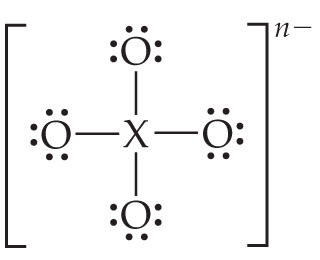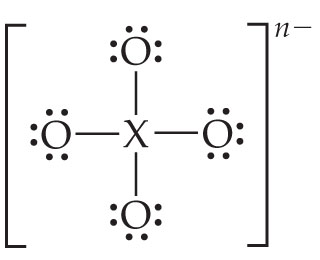# Problem: Consider the Lewis structure for the polyatomic oxyanion shown here, where X is an element from the third period (Na-Ar). By changing the overall charge, n, from 1- to 2- to 3- we get three different polyatomic ions.For each of these ions draw a Lewis structure that makes the formal charge on the central atom equal to zero.

###### FREE Expert Solution

We are asked to draw a Lewis structure that makes the formal charge on the central atom equal to zero

We are given that X is an element from the third period (Na-Ar). By changing the overall charge, n, from 1- to 2- to 3- we get three different polyatomic ionsTo do so, we need to do the following steps:

Step 1: Determine the number of valence electrons and the identity of X.

Step 2: Determine the formal charge of X.

Step 3: Draw the Lewis structure for the molecule.

Step 4: Draw the Lewis structure that makes the formal charge on the central atom equal to zero.

Recall that the formula for the formal charge is:

84% (489 ratings)###### Problem Details
Consider the Lewis structure for the polyatomic oxyanion shown here, where X is an element from the third period (Na-Ar). By changing the overall charge, n, from 1- to 2- to 3- we get three different polyatomic ions.For each of these ions draw a Lewis structure that makes the formal charge on the central atom equal to zero.